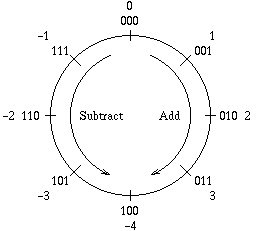# Two's Complement Cheatsheet

Quick conversion rules.

### Binary Positive to Negative

Invert all the bits and add one:

```0101    =>    5

1010
+  1
----
1011    =>   -5
```

### Binary Negative to Positive

Invert all the bits and add one:

```1011    =>   -5

0100
+  1
----
0101    =>    5
```

### Binary Negative to Decimal

Consider the highest-order bit as having a negative weight and all other bits as having a positive weight:

```1011    =>   -1*2^3 + 0*2^2 + 1*2^1 + 1*2^0

=>   -8 + 0 + 2 + 1

=>   -5
```

### Unsigned Complements

Thinking in terms of unsigned n-bit integers, the two's complement of `x` is always given by `2^n - x`. For example, for 4-bit numbers:

```2^4 - 5    =    16 - 5    =    11    

2^4 - 11   =    16 - 11   =     5    
```

In a 4-bit world the two's complement of 5 is 11 and the two's complement of 11 is 5. A number and its complement always sum to `2^n`.

### Range

An n-bit two's complement integer can represent the range from –2n-1 to 2n-1 – 1. For example, a 3-bit two's complement integer can represent the range from –22 to 22 – 1, i.e. from –4 to 3, as illustrated below: